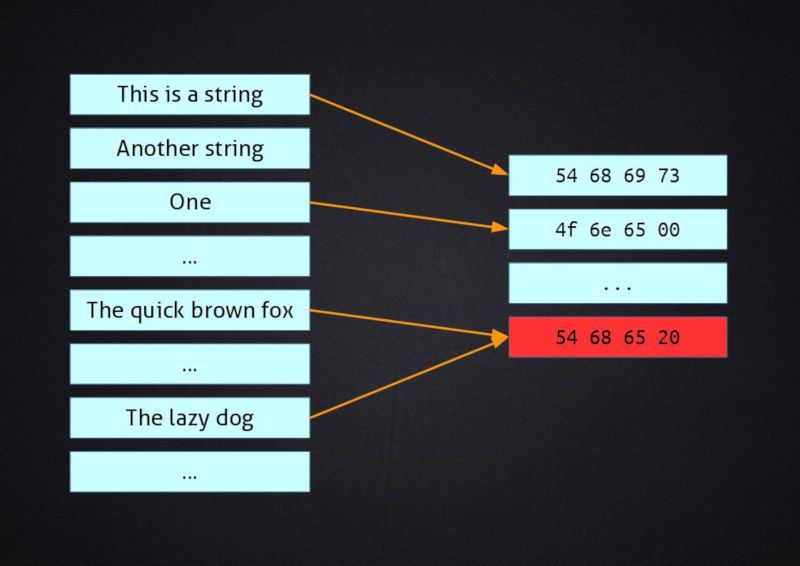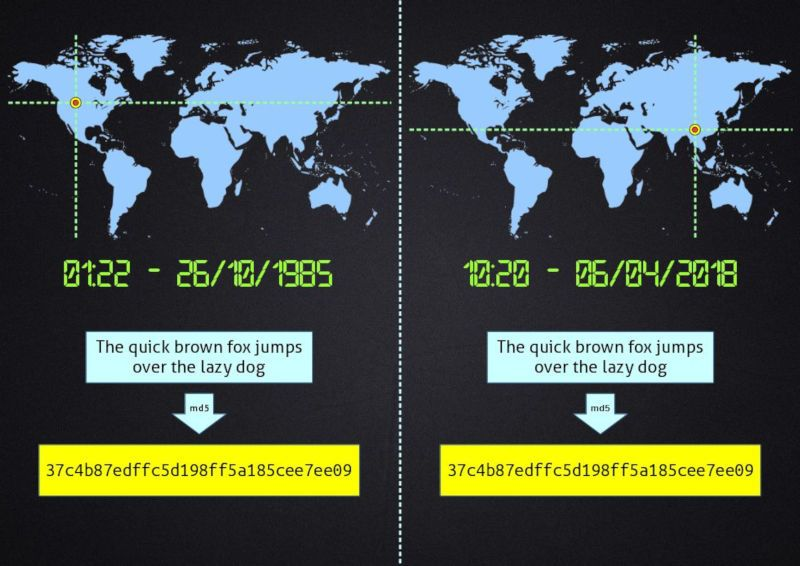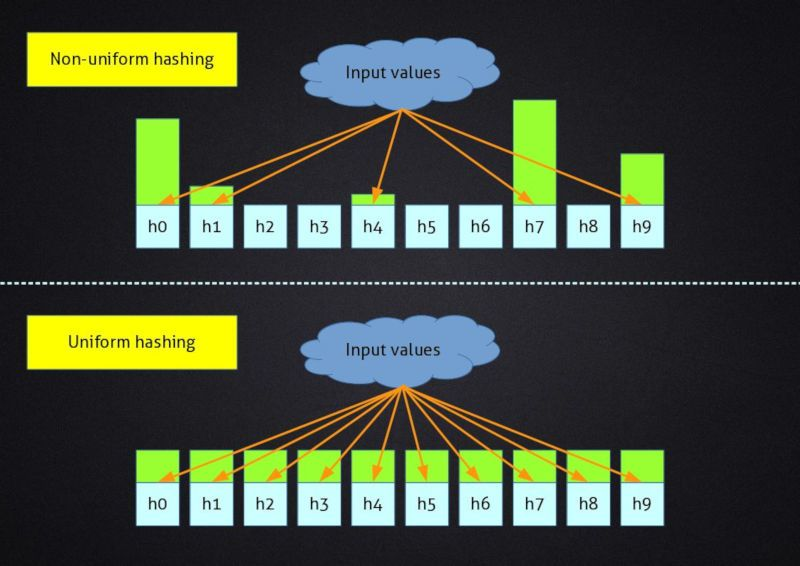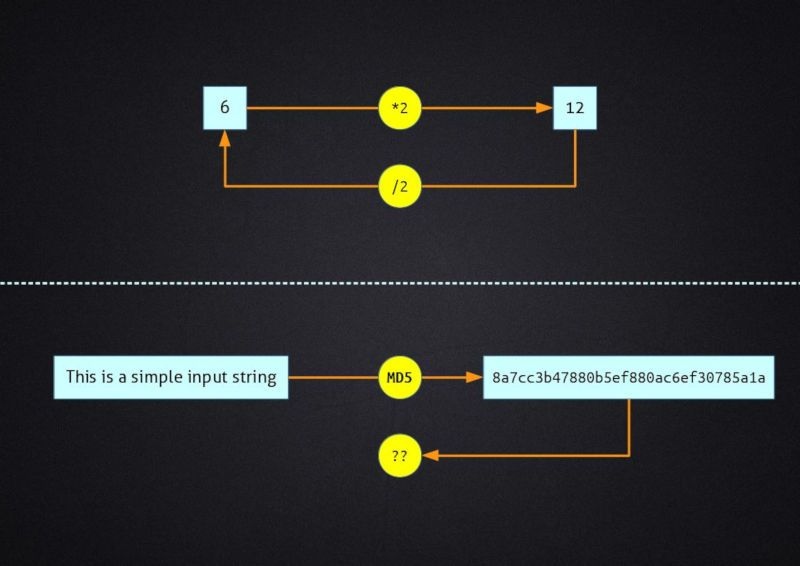By Leonardo Giordani Published on

Have you ever used dictionaries or maps in your language of choice, or have you ever met a mysterious MD5 code while downloading a file from a server? Maybe you are a programmer, and using Git to manage your code you ended up dealing with strange numbers called SHA1, and surely you heard at least a couple of times the term cache, which probably needed to be emptied in your browser.

What do all these concept have in common?

In this post I want to introduce you to the concept of hashing, which is one of the basic topics a good programmer shall know. Hashes are such an important topic in computer science that lacking knowledge in this field means being confused about a wide range of other subjects, like cryptography and security. Just to name some of the players you could be interested in your future career as a programmer: data structures, Bitcoin and blockchains, HTTPS. All these topics have hashing as one of their building blocks. As you can see, it is worth mastering the concept.

This will obviously be only a humble introduction to the subject matter, as the whole concept is too broad for a single post. You can start a serious study of this important part of computer science reading the Wikipedia articles liked at the bottom of the page and reading a good book (or taking a course) in either cryptography or data structures.

# A practical example¶

Let me give you a concrete example of hashing before we analyse the matter in depth.

I want to download a big file from Internet, like a Linux distribution. I already downloaded it in the past, but I'm not sure if the version I have is the same available for download now. The file has been renamed, so the original name has been lost. I might obviously just download it again, install it and manually check the version.

I wonder if there is a simpler solution, one that can possibly be automated. Downloading an ISO file from Internet is nowadays very cheap, but manually checking for the version isn't, at least in terms of time. I might also download it and compare all the files contained in both the new and the old ISO images, but then again, this process is not very fast.

The best solution would be for the server to provide a sort of label that depends only on the data in a mathematical and deterministic way. I might then run the same algorithm on the file I already downloaded and get a label that can be easily compared with the one provided by the server. If the process of computing the label is fast enough this might be the perfect solution.

A typical algorithm used for this purpose is MD5, and the label computed by the server could be something like `ef67d799b71de37423202c587662c87f`. Computing the MD5 of a 600 MB file takes less than a couple of seconds on a modern computer, so I can check if the file I own is the same the server provides in a very short time.

You can test MD5 on your own using the `md5sum` program that comes with all Linux distributions or other Unix-based systems. Open a terminal and run the following command

```echo "This is a simple input string" | md5sum
```

and the result will be `8a7cc3b47880b5ef880ac6ef30785a1a`, independently of your operating system.

MD5 is one of many hash functions that have been invented to deal with problems like the one I exemplified. Recently I had the need to synchronise daily two AWS S3 buckets containing more than 60 gigabytes of files. Without hash functions it would be impossible to quickly identify the files that need to be copied.

The rest of the post is dedicated to the exploration of such an important and intriguing part of contemporary technology.

# Hash functions¶

Let's start from the formal definition of hash function:

A hash function is any function that can be used to map data of arbitrary size to data of fixed size

This description may sound intimidating at first, but it is actually pretty simple. Let's consider a dictionary where you want to look up a word that you don't know, like for example "quagmire". What you do is to jump directly to a section labelled "Q" in the dictionary, then quickly identify the part of the section where words that start with "QU" are, and promptly find the word. Congratulations, you just used a hash function!Getting the first letter of the word is, as a matter of fact, a function (an operation) that maps (connects) data of arbitrary size (words) to data of fixed size (a single letter of the alphabet). Using this method we can connect any word (also invented ones!) to a letter of the alphabet.

Before we move on, I want to stress one aspect that is clear from the previous example. Through a hash function we can connect a set of potentially infinite values (all the words that we can create) with a finite set (the letters of an alphabet). This is the most important concept we have to keep in mind when dealing with hashing.

# Uniqueness¶

The result of a hash function is not unique, which means that two different inputs may give the same output. This is pretty easy to understand in the dictionary example, where multiple words can give as a result the same letter, as multiple words begin with that letter.

It is also evident both from the dictionary example and from the definition that hash functions cannot produce unique results by design. The goal of a hash function is to map an infinite set with a finite set, so it is obvious that multiple elements of the infinite set will map to the same element in the finite one.

Let me give you a very simple example. Let's create a hash function that returns the first 32 bits (4 bytes) of the input, padding them with zeros if the input is shorter that 32 bits. I will use the ASCII standard to convert strings of characters into hexadecimal numbers, so every letter is represented by 1 byte.

```"This is a string" == 54 68 69 73 20 69 73 20 61 20 73 74 72 69 6e 67 => 54 68 69 73
"One" == 4f 6e 65 =>  4f 6e 65 00
"The quick brown fox" == 54 68 65 20 71 75 69 63 6b 20 62 72 6f 77 6e 20 66 6f 78 => 54 68 65 20
"The lazy dog" == 54 68 65 20 6c 61 7a 79 20 64 6f 67 => 54 68 65 20
```

As you can see we have multiple input strings with different lengths, and while the first three produce different output values the last one produces the same value as the third one. This is straightforward, as the two strings start with the same four characters and our hash function considers only those to compute its result.

Such an event is called collision and it is a direct effect of the non-uniqueness of hash values. It will always happen, with hash functions, that different values produce the same output, and it is important to understand that this is not because our hash function is trivial, but this is in the very nature of hash functions, for strict mathematical reasons.

Collisions are not intrinsically bad, but we have to be aware they can happen when we develop algorithms that use hash functions. If we are writing a dictionary for a human language where 80% of the words starts with "A" it is pointless to use the first letter to partition the book because the first section would be almost as big as the whole tome. This may seem too imaginative an example, but when we manage data structures problems such this arise at every corner.In this last example avoiding collisions is easy. We just need to increase the number of characters that we consider until the size of the book sections becomes reasonable. When the the hash function becomes more complex, especially when it has the diffusion property (explained later), avoiding the clustering in the output data might not be so simple.

# Digital hash functions¶

As we saw the definition of hash functions involves functions, which are mappings. In other words we just need to describe a process that couples objects from the source infinite set to the destination finite set. Taking the fist letter of a word is such a process, but other examples may be grouping people according to the colour of the eyes or cataloguing films by production year. Among the various processes that we can use a big role is played by digital processes, that is functions that involve some operation on binary numbers.

When we digitalise something we represent it with a sequence of bits, and once this is done there is no real difference between strings, images, videos, sounds, programs. Everything in a computer is ultimately a sequence of bits, and those sequences can be sliced and changed with pure numerical functions such as additions and multiplications.

# Cryptographic hash functions¶

Hash functions play a decisive role in security and in cryptography, and they can mostly be found in algorithms that provide authentication, i.e. secure ways to demonstrate the authenticity of some data. While the actual cryptographic techniques are not in the scope of this article, it is important to know that hash functions used for cryptographic purposes are not different from hash functions used for other tasks that do not require any degree of security. Cryptographic hash functions, however, must have some specific properties that give the function a certain degree of "robustness". Being able to find the input of a hash function given the output, for example, would be catastrophic for some security algorithms that rely on the infeasibility of such an operation.

# "Good" hash functions¶

While the definition of hash function is pretty inclusive, stating that the only required property is that of returning a fixed-length output, hash functions used in practice may have other properties. Such properties may be desirable or mandatory depending on the application, so functions that are extremely good for cryptography may be a poor choice as a base for data structures like dictionaries.

Let me briefly describe some of the most important ones that you should be aware of.

## Determinism¶

Given the algorithm (with its parameters), the hash number of some data must always be the same. The result of the hashing function depends only on the data itself, and not on other external factors like for example time or computer system.

Pay attention to the fact that this definition considers the algorithm and its parameters. This means that we can include external factors in the computation, but they have to be fixed for the whole life of the result itself.Let's consider a system that uses a hash to speed up searches in some arrays. For several reasons the hashing algorithm employs an initial random seed that is derived from the boot time. As long as the system is running (i.e. it is not rebooted), the algorithm is consistent, and we may consider the random seed as a constant parameter. We may also persist the hashes on a storage, because when we load them they are still perfectly valid. As soon as the system is rebooted, however, the whole set of hashes created during the previous execution becomes invalid and meaningless. This is not the case, though, if the hashing function bases its computation on the actual data only.

## Diffusion¶

Changing one single bit of the source data shall results in a complete change of the hash number. Compare for example the MD5 hash values of two similar strings

```The quick brown fox jumps over the lazy dog => 37c4b87edffc5d198ff5a185cee7ee09
The quick brown fox jumps over the lazy cog => 15546a0bcace46fd5e12ec29adca5e70
```

As you can see when a single input byte is different (the letter `d` becomes a `c`), the whole result changes.

This implies that every part of the hash function's output is computed considering all the bits of the input. A function that returns the first `n` bits of the input does not have a good diffusion, as two different strings may return exactly the same hash if they have the same first `n` bits (see the example given above when I spoke about uniqueness). This property is important for cryptographic hash function.

## Minimal change (continuity)¶

An interesting property of some hash functions is that similar input values map to similar hash values. The exact definition of "similar" may vary, but in general we might associate it with the number of changes from the first output to the second. This behaviour is handy in some searching algorithms, where it is important that similar objects are stored near each other.

Note that this property is somehow the opposite of diffusion, thus demonstrating that not all these properties might be found in a single hash function.

## Uniformity¶

A hash function has a given finite number of possible outputs, because the output has a finite length. When a hash function is uniform, producing the output for each possible input produces a uniform distribution of outputs, that is there is no output value that is used more often than others. When designing data structures this is often the desirable behaviour, since it leads to an uniform use of resources, for example memory, leading to an uniform behaviour of other algorithms that work on the same structure, like search.Uniformity is obviously linked to the number of collisions produced by a hash function, and a perfectly uniform hash function will have the same number of collisions for each output value. Increasing the number of possible output values, thus, results in a uniform reduction of collisions.

## Non-invertible¶

Inverting a function means to create a function that returns the original input given the output. For example multiplication by 2 is an invertible function, as given the result we may easily divide by 2 and retrieve the original input.

With non-injective functions the only caveat is that there are multiple inputs that return the same output, but this doesn't prevent the creation of an inverse function. For example, 3 squared gives 9 and since the inverse of the square function is the square root, we can apply it to the result and retrieve the possible inputs, that is +3 and -3.

With non-invertible functions there is no simple way to find the input given the output. Mathematically we speak of one-way functions, as computing the inverse is either impossible of infeasible. Mind that "infeasible" has a well-defined meaning in mathematics, but I will not go deeper into it in this article. It will be sufficient to consider it as "too hard to compute in a reasonable time with the current state of technology". Cryptographic hash functions must be non-invertible.## Collisions-resistant¶

A hash function is said to be collision-resistant when it is hard to find two different inputs that produce the same hash value. Mind that the definition of "hard" here is the same as that of "infeasible" in the previous section. This property is very important in cryptography, where collisions can be exploited to crack a cipher.

# Theoretical and practical inputs¶

It is important to understand that the analysis of a hash function can be made considering either theoretical or practical inputs. Theoretical inputs are all the possible inputs, like "all the possible strings", while a set of practical inputs might be "the names of a group of people". The latter might be very large but it is not infinite.

Obviously, a hash function that provides interesting properties when dealing with theoretical inputs will show the same properties when applied to practical inputs, but often such functions are complex and slow. Not to mention that it is very difficult to create them.

Let me show you an example. As we saw above, a hash function that returns the first letter of a string is not a very good one. It lacks the diffusion property, for instance, an its uniformity is questionable, as all the strings that begin with the same letter will produce the same hash, leading to a large number of collisions. This is bad for data structures, so such a function is in theory not optimal.

However, if we are working on a set of strings like

```A poor workman blames his tool
Barking dogs seldom bite
Common sense ain't common
Doctors make the worst patients
...
You can't teach an old dog new tricks
```

where it is known (or evident) that each string begins with a different letter, suddenly our hash function becomes a perfect choice to build a searchable data structure, because given this input set there are no collisions. So, an analysis of the practical inputs is always paramount when we consider hash functions, as theoretically poor functions may perform very well on specific sets of inputs.

A very good example of such an analysis can be found in the source code of the Python language. The implementation of dictionaries contains an in-depth discussion of the choices made when implementing the hashing mechanism behind those structures. You can find it here. If you never approached data structures I recommend starting from a simpler explanation, however, as you might be intimidated by that discussion. You will find a good basic tutorial on hash tables in any data structures course or textbook.

# Final words¶

As I said this is just a very quick and humble introduction to hashing. I think you cannot call yourself a programmer nowadays without knowing something about hashing, and what I summarized in this post is enough to understand hash uses like Bitcoin or SSL. If you want to study the topic in depth, however, I recommend taking a course or reading a book on data structures.

# Resources¶

2018-04-28 gixslayer and SevenGlass discussed on reddit the right command line for the `md5sum` example on Windows. See https://www.reddit.com/r/programming/comments/8fbepo/introduction_to_hashing/dy316go